# Orthogonal sets

### Orthogonal sets

#### Lessons

A set of vectors {$v_1,\cdots,v_n$} in $\Bbb{R}^n$ are orthogonal sets if each pair of vectors from the set are orthogonal. In other words,
$v_i \cdot v_j =0$
Where $i \neq j$.

If the set of vectors {$v_1,\cdots,v_n$} in $\Bbb{R}^n$ is an orthogonal set, then the vectors are linearly independent. Thus, the vectors form a basis for a subspace $S$. We call this the orthogonal basis.

To check if a set is an orthogonal basis in $\Bbb{R}^n$, simply verify if it is an orthogonal set.
$y=c_1 v_1+c_2 v_2+\cdots+c_p v_p$

Are calculated by using the formula:
$c_i = \frac{y \cdot v_i}{v_i \cdot v_i}$
where $i=1,\cdots,p$.

A set {$v_1,\cdots,v_p$}is an orthonormal set if it's an orthogonal set of unit vectors.

If $S$ is a subspace spanned by this set, then we say that {$v_1,\cdots,v_p$} is an orthonormal basis. This is because each of the vectors are already linear independent.

A $m \times n$ matrix $U$ has orthonormal columns if and only if $U^T U=I$.

Let $U$ be an $m \times n$ matrix with orthonormal columns, and let $x$ and $y$ be in $\Bbb{R}^n$. Then the 3 following things are true:
1) $\lVert Ux \rVert = \lVert x \rVert$
2) $(Ux) \cdot (Uy)=x \cdot y$
3) $(Ux) \cdot (Uy)=0$ if and only if $x \cdot y =0$

Consider $L$ to be the subspace spanned by the vector $v$ . Then the orthogonal projection of $y$ onto $v$ is calculated to be:
$\hat{y}=$proj$_Ly=\frac{y \cdot v}{v \cdot v}v$

The component of $y$ orthogonal to $v$ (denoted as $z$) would be:
$z=y-\hat{y}$
• Introduction
Orthogonal Sets Overview:
a)
Orthogonal Sets and Basis
• Each pair of vector is orthogonal
• Linear independent → Form a Basis
• Calculate weights with Formula

b)
Orthonormal Sets and Basis
• Is an orthogonal set
• Each vector is a unit vector
• Linear independent → Form a Basis

c)
Matrix $U$ with Orthonormal columns and Properties
$U^T U=I$
• 3 Properties of Matrix $U$

d)
Orthogonal Projection and Component
• Orthogonal Projection of $y$ onto $v$
• The component of $y$ orthogonal to $v$

• 1.
Orthogonal Sets and Basis
Is this an orthogonal set?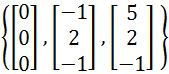• 2.
Verify that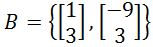is an orthogonal basis for $\Bbb{R}^2$, and then express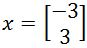as a linear combination of the set of vectors in $B$.

• 3.
Orthonormal Sets/Basis
Is set $B$ is an orthonormal basis for $\Bbb{R}^3$?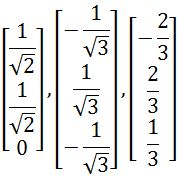• 4.
Let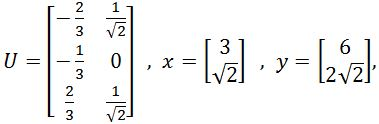where $U$ has orthonormal columns and $U^TU=I$. Verify that
$(Ux)\cdot (Uy)=x\cdot y$

• 5.
Orthogonal Projection
Let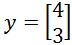and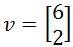. Write $y$ as the sum of two orthogonal vectors, one in Span{$v$} and one orthogonal to $v$.